# Choosing the Right Center of Projection For Your Map

Each projected map should make the feature at hand the center of projection.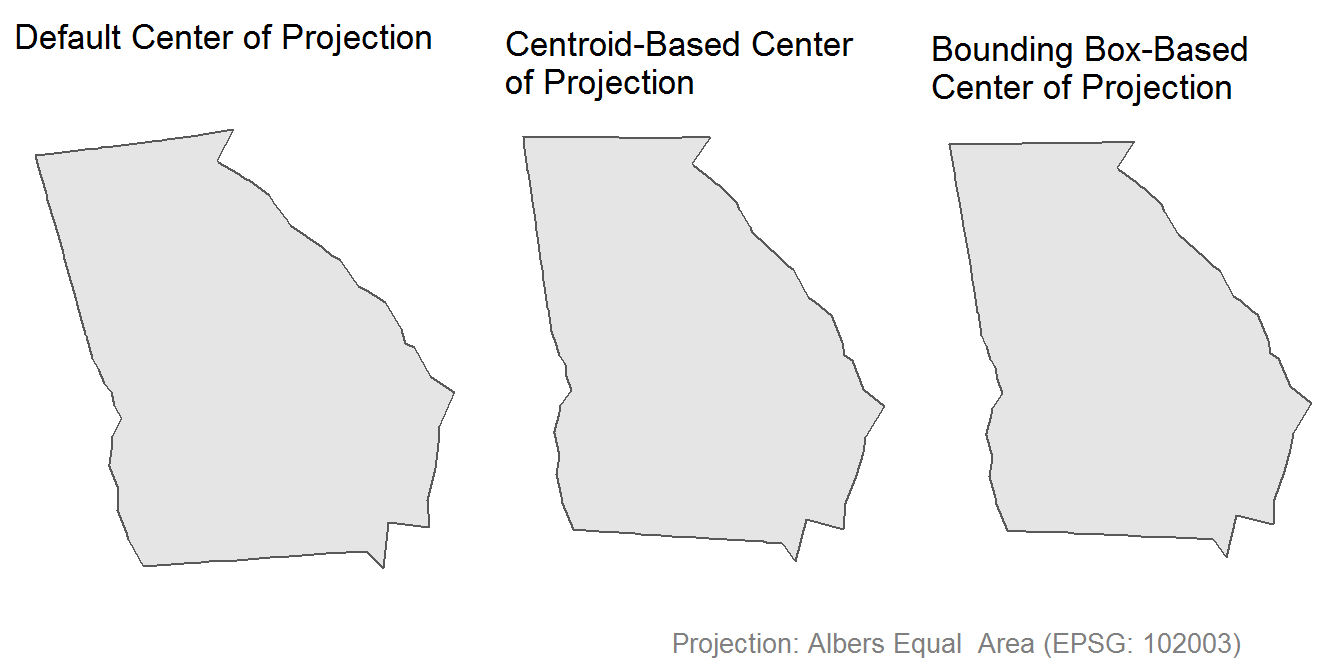The following map of Georgia comes from the Washington Posts’ coverage of the 2018 primary elections.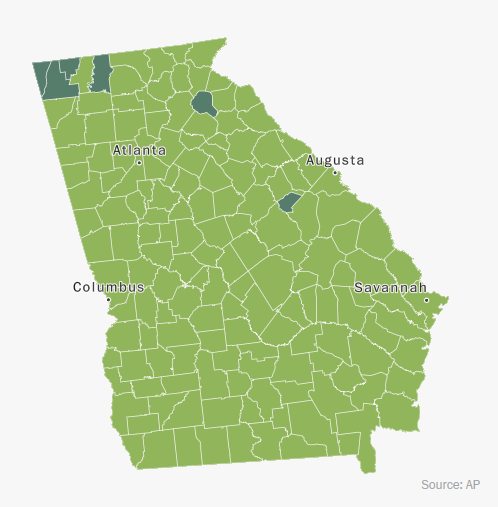These kinds of maps - where the center of projection is not based on the feature being mapped - are seen quite often in the Washington Post, New York Times, and other major US news publishers.

The map at hand shows the state of Georgia - tilted to the left, as though rotated counter-clockwise about its center. Why is that?

## DefaultsThe map-making software used by the Washington Post is doing one of two things:

• Preserving the center of the contiguous 48 states
• Preserving some other default center of projection

NOTE: I do not know which software they use.

Here are two maps to help explain what is going on.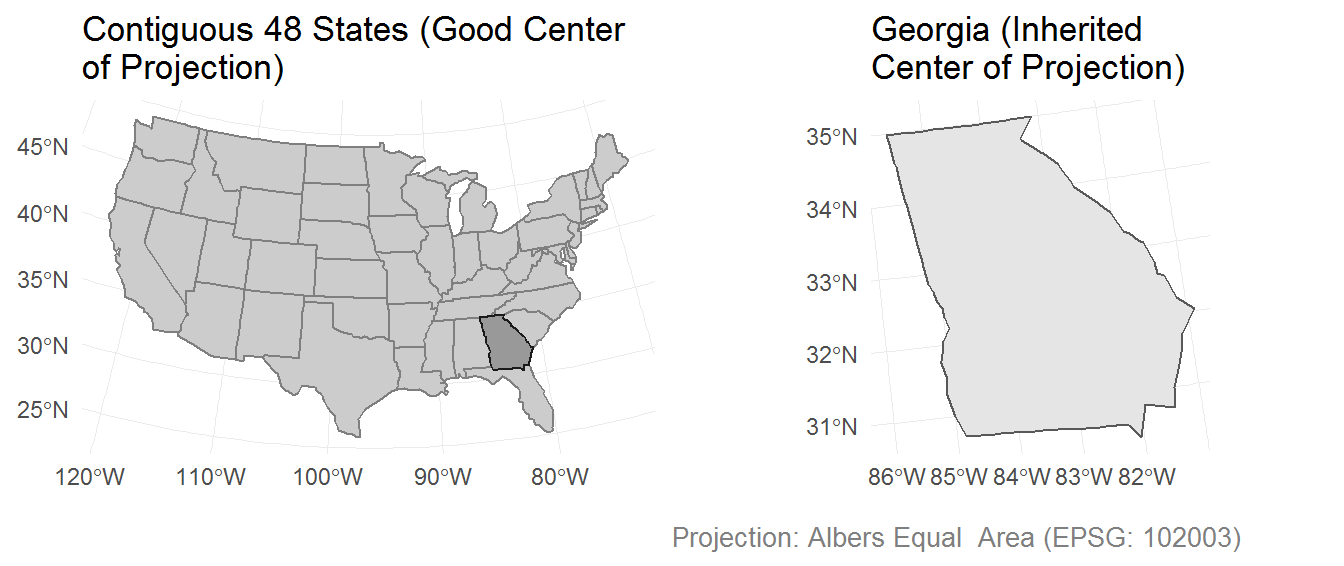## Center of Projection

The center of the projection is defined by the latitude of origin and the central meridian - `lat_0` and `lon_0` within a proj4string, respectively. A proj4string describes a CRS (coordinate reference system) through the use of arguments and and their respective values.

The above map of the US and Georgia uses a proj4string generated by `st_crs(102003)`, from the R package `sf`. That function is passed to a transformation function, to tell it which projection to produce.

The central meridian (`lon_0`) produced by `st_crs(102003)` is suitable for projecting the contiguous 48 states (it uses NAD83 datum).

``````st_crs(102003)
#> Coordinate Reference System:
#>   EPSG: 102003
#>   proj4string: "+proj=aea +lat_1=29.5 +lat_2=45.5 +lat_0=37.5 +lon_0=-96 +x_0=0 +y_0=0 +datum=NAD83 +units=m +no_defs"``````

## Solutions

### Finding an Appropriate Center of ProjectionFinding a new center of projection is not so difficult, and I have two methods in mind to demonstrate.

For the following examples, I will be using:

#### Centroid

This approach uses the coordinates of an unprojected centroid as the center of projection.

These coordinates can be plugged in as values to the appropriate proj4string arguments.

``````us %>%
filter(state == "GEORGIA") %>%
st_centroid() %>%
st_coordinates()
#>           X        Y
#> 1 -83.44397 32.64748

us %>%
filter(state == "GEORGIA") %>%
st_transform(crs = "+proj=aea +lat_1=29.5 +lat_2=45.5 +lat_0=32.6 +lon_0=-83.4 +x_0=0 +y_0=0 +datum=NAD83 +units=m +no_defs") %>%
ggplot() +
geom_sf() +
theme_minimal()``````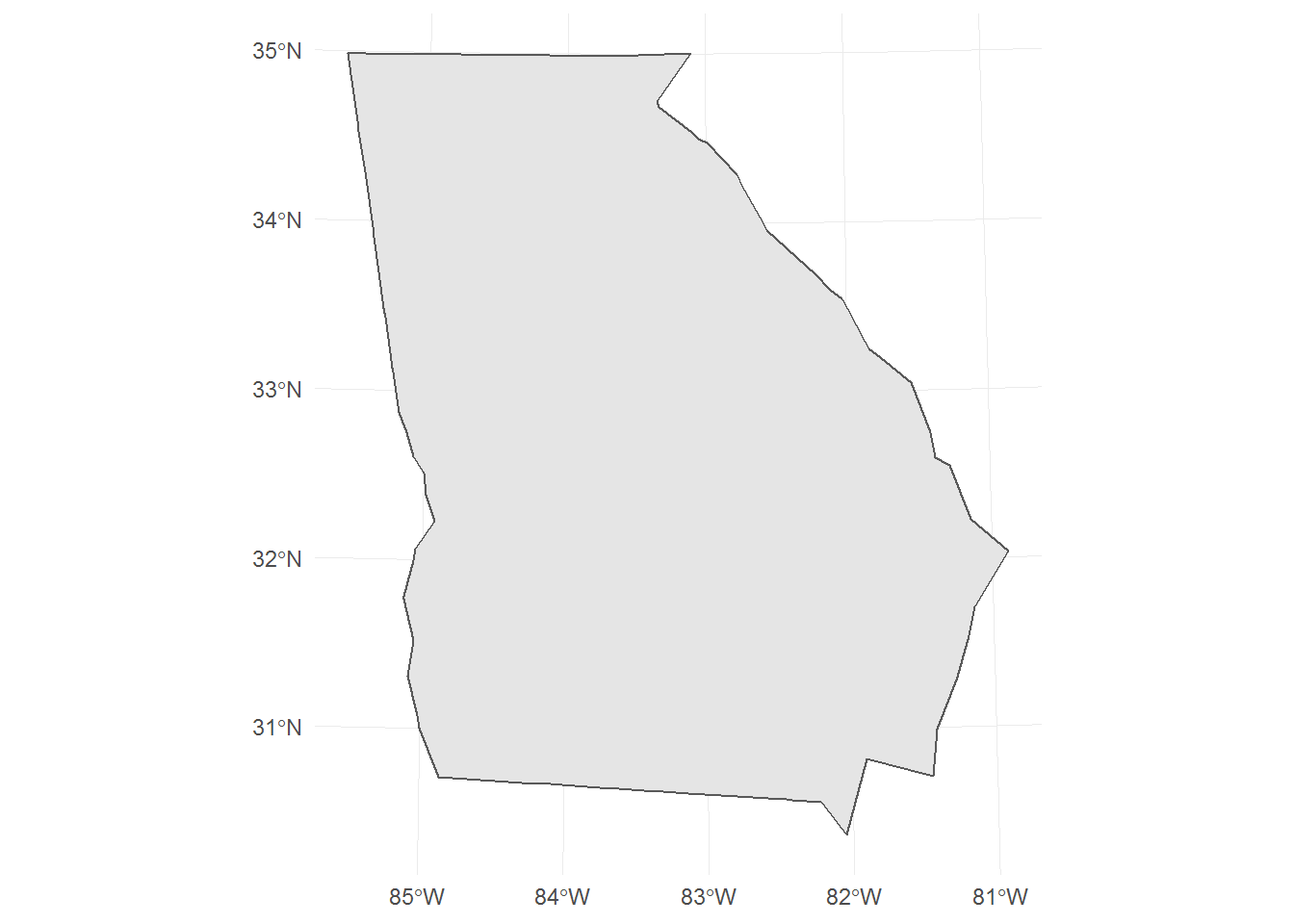#### Bounding Box

The bounding box provides another value from which to extract a center of projection.

``````us %>%
filter(state == "GEORGIA") %>%
st_bbox() %>%
{
c(x = mean(.\$xmin, .\$xmax),
y = mean(.\$ymin, .\$ymax))
}
#>         x         y
#> -85.60516  30.36837

us %>%
filter(state == "GEORGIA") %>%
st_transform(crs = "+proj=aea +lat_1=29.5 +lat_2=45.5 +lat_0=30.3 +lon_0=-85.6 +x_0=0 +y_0=0 +datum=NAD83 +units=m +no_defs") %>%
ggplot() +
geom_sf() +
theme_minimal()``````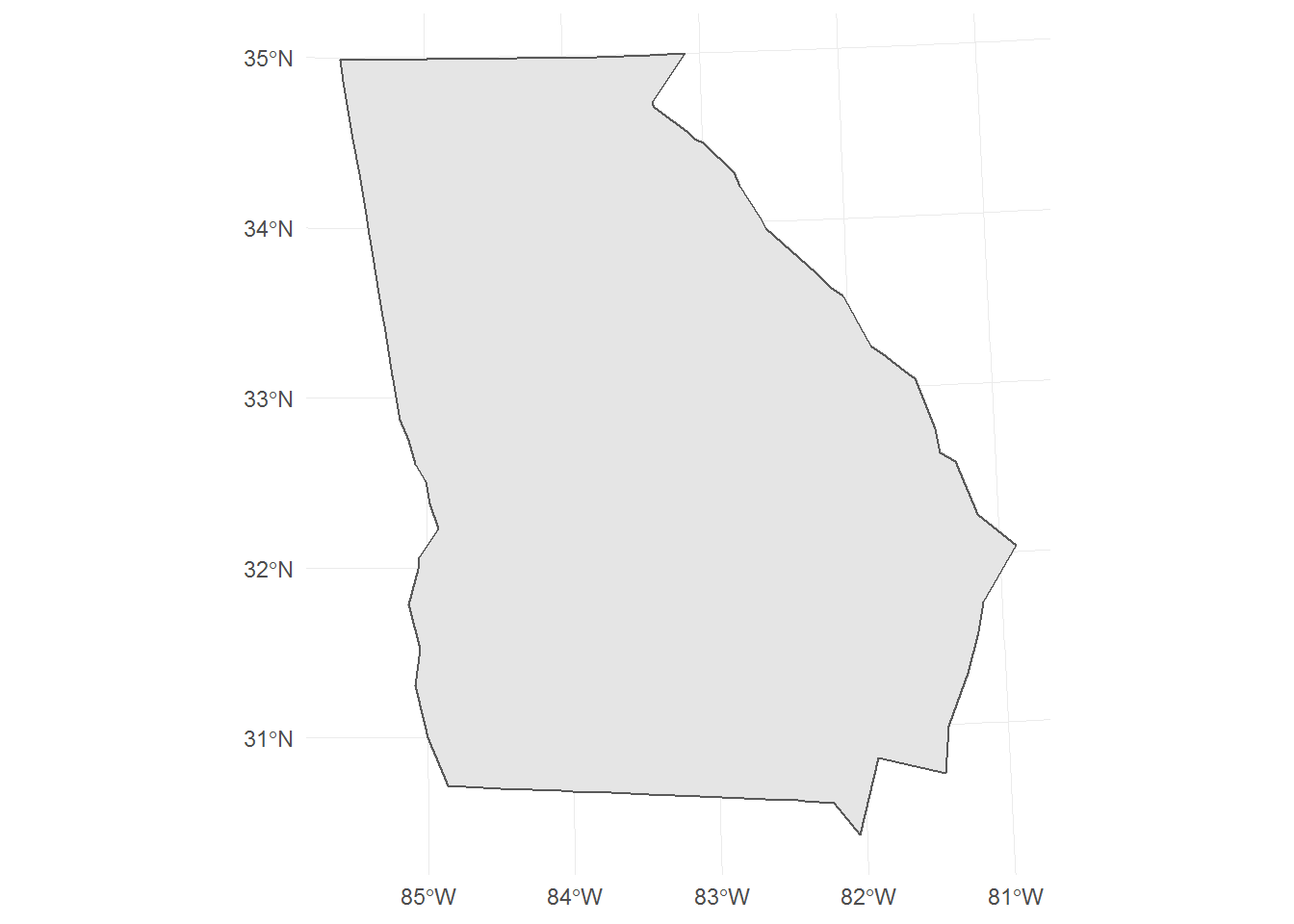### A Different Projection

I don’t know which projection the Washington Post used in their map of Georgia, but it looks like either an Albers Equal Area or a Lambert Azimuthal Equal Area (EPSG: 2163).

These may not always be the best projections to use, especially when mapping such small regions.

#### World Mercator

This projection produces right-angle graticules (lines of latitude and longitude) - preserving directionality (i.e. east is always a straight-line to the right).

Essentially, you can ignore the center of projection when using this projection. It is a good projection for areas outside of the high-latitudes (where area distortion does occur).

``````us %>%
filter(state == "GEORGIA") %>%
st_transform(crs = st_crs(3395)) %>%
ggplot() +
geom_sf() +
theme_minimal()``````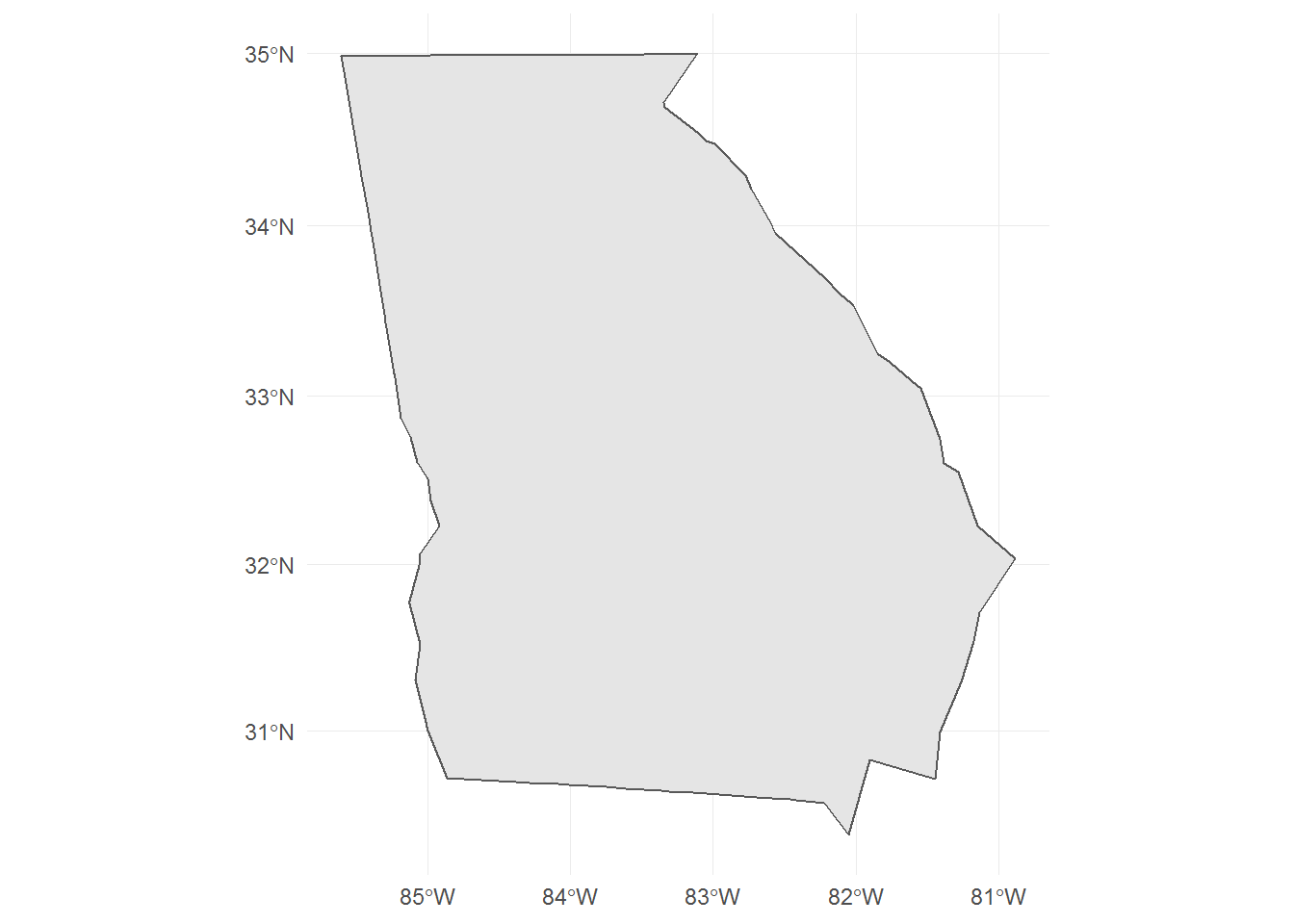For unprojected coordinates, not specifying a projection is almost akin to using a World Mercator.

``````us %>%
filter(state == "GEORGIA") %>%
ggplot() +
geom_sf() +
theme_minimal()``````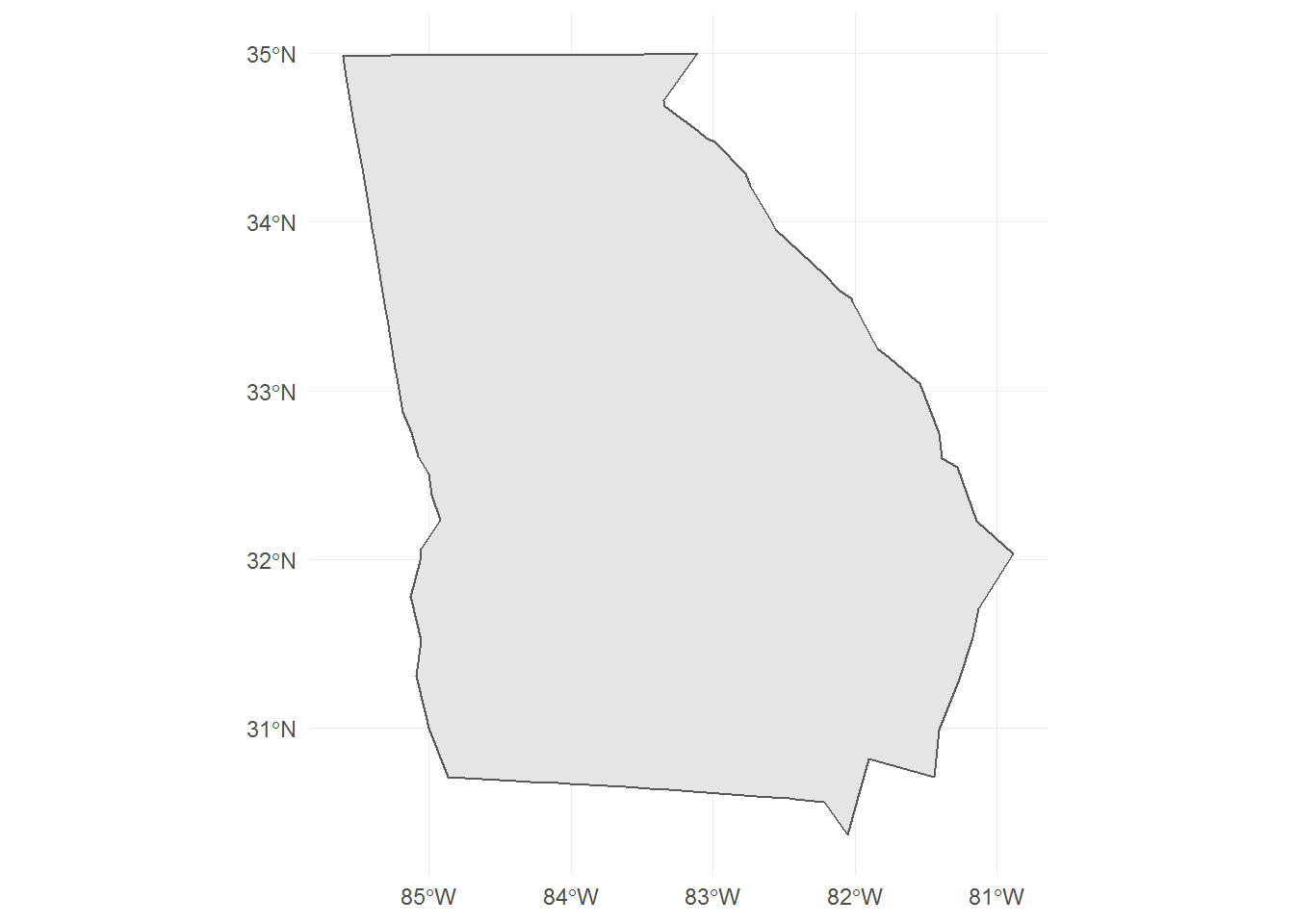## When Not to Center

Maybe there are times when using an inherited center of projection is appropriate.

One such case is when two maps are placed side-by-side, with one map being a subset of the other. In such a case, you may want to preserve the orientation of the subsetted feature in side-by-side map.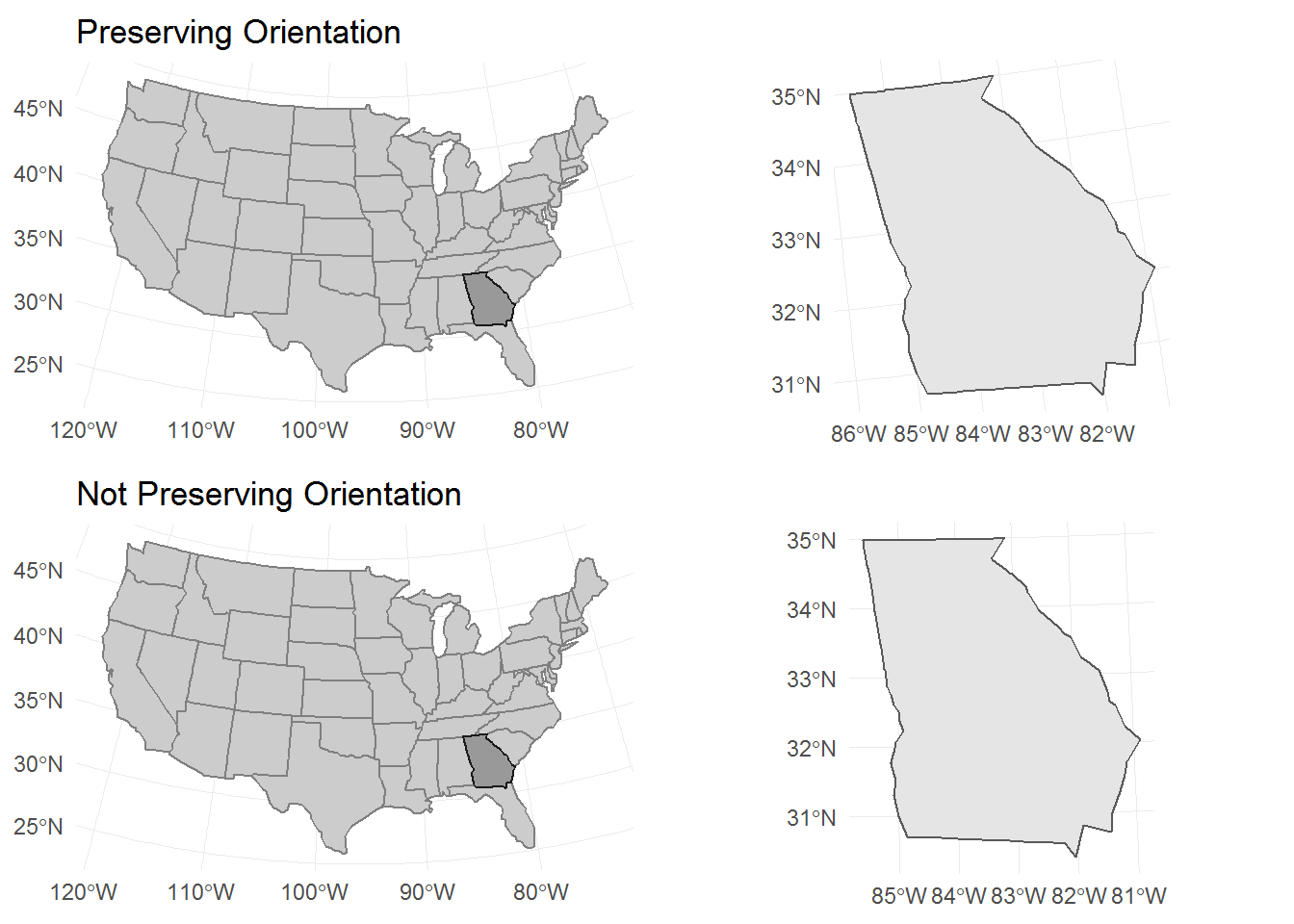## Long-term Solution

Many GIS programs, like `sf`, do not re-center subsetted features (to preserve the CRS across related geometries). This means that the user will have to manually perform this task.

This may involve saving a custom proj4string for each known subsetted feature, and loading using that file when creating a map.

Published by and tagged ggplot2, gis, map-projections, r and sf using 679 words.